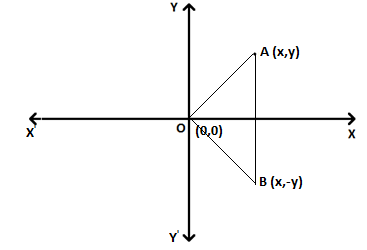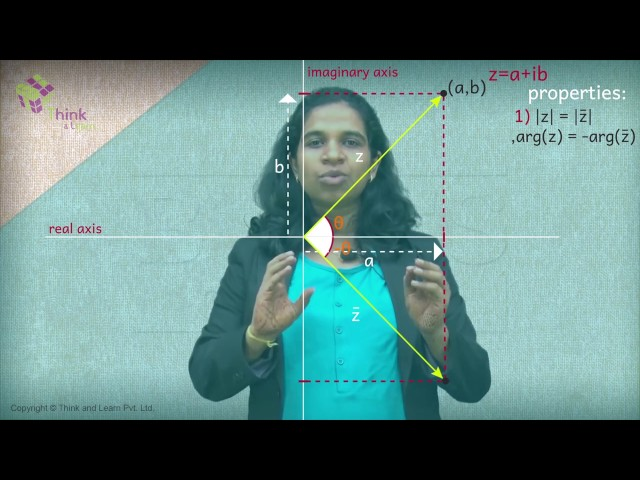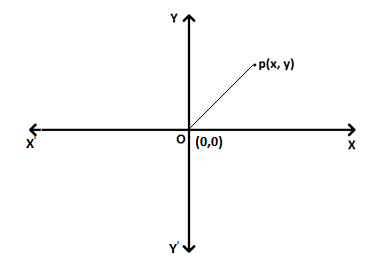# Conjugate of Complex Number

## Operations on Complex Numbers

We can perform several algebraic operations on complex numbers, such as addition, multiplication, division, etc. However, we can find some interesting parameters for any given complex numbers using certain operations. Let’s learn how to find the conjugate and modulus of complex numbers here with help of formulas, examples, and a video lesson.

## Conjugate of a Complex Number

Conjugate of a complex number z = x + iy  is x – iy and which is denoted as

$$\begin{array}{l}\overline{z}.\end{array}$$

For example, the conjugate of the complex number z = 3 – 4i is 3 + 4i.

Consider the complex number z = a + ib. For this, we can define the following formulas.

$$\begin{array}{l}z + \overline{z}=a + ib + (a – ib)=2a\end{array}$$
which is a complex number having imaginary part as zero.

Here,
$$\begin{array}{l}Re(z + \overline{z})=2a, Im(z + \overline{z}) = 0\end{array}$$
• Subtraction:
$$\begin{array}{l}z – \overline{z}=a +ib – (a – ib)=2bi\end{array}$$

Here,
$$\begin{array}{l}Re(z – \overline{z})=0, Im(z -\overline{z})=2b\end{array}$$
• Geometrically, reflection of the complex number z = x + iy in x-axis is the coordinates of
$$\begin{array}{l}\overline{z}.\end{array}$$#### Video Lesson## Modulus of Complex Number

Let z = x + iy be a complex number, modulus of a complex number z is denoted as |z| which is equal to √(x2 + y2).

Geometrically, the modulus of a complex number z = x + iy is the distance between the corresponding point of z which is (x, y) and the origin (0, 0) in the argand plane.In the above figure, OP is equal to the distance between the point (x, y) and origin (0, 0) in the argand plane.

Therefore, |z| = OP = √(x2 + y2).

Example:

Find the value of b if the modulus of the complex number, z = 3 + ib is equal to 5.

Solution:

Given,

Z = 3 + ib

|z| = √(32 + b2)

√(9 + b2) = 5 (from the given)

9 + b2 = 25

b2 = 25 – 9

b = √16

Thus, b = ±4.

From the above example, we can conclude that complex numbers z1 = x + iy = 3 + 4i and z2 = x – iy = 3 – 4i will have same modulus which is equal to √(x2 + y2). It is because the points corresponding to the above four complex numbers(x, y), (x, -y), (-x, y) and (-x, -y) respectively are at a distance √(x2 + y2) away from origin.

Properties

• The modulus of the complex number and its conjugate will be equal.
• Multiplicative inverse of the non-zero complex number z = a + ib is
$$\begin{array}{l}z^{-1}=\frac{1}{a+ib}=\frac{a-ib}{a^2+b^2}\end{array}$$
• As we know,
$$\begin{array}{l}\overline{z} = a – ib\end{array}$$
and
$$\begin{array}{l}|z|^2=a^2+b^2\end{array}$$
.
• $$\begin{array}{l}z^{-1}=\frac{z}{|z|^2}\end{array}$$
• $$\begin{array}{l}z\overline{z}=|z|^2\end{array}$$

Example:

Find the multiplicative inverse of z = 6 + 8i.

Solution:

Given,

z = 6 + 8i

So,

$$\begin{array}{l}\overline{z} = 6 – 8i\end{array}$$

|z| = √(62 + 82) = √(36 + 64) = √100 = 10

We know that

$$\begin{array}{l}z^{-1} = \frac{\overline{z}}{|z|^2}\end{array}$$

Thus, z-1 = (6 – 8i)/(10)2 = 2(3 – 4i)/100 = (3 – 4i)/50 = (3/50) – (2/25)i

### Identities of complex numbers

For any two complex numbers z1 and z2,

|z1 z2| = |z1| |z2|

We can prove this identity as follows.

Let z1 = a + ib and z2 = c + id

|z1| = √(a2 + b2)….(1)

|z2| = √(c2 + d2)….(2)

Now,

z1z2 = (a + ib)(c + id) = (ac – bd) + (bc + ad)i

|z1z2| = √[(ac – bd)2 + (bc + ad)2]

|z1z2|2 = (ac – bd)2 + (bc + ad)2

= a2c2 + b2d2 – 2abcd + b2c2 + a2d2 + 2abcd

= a2c2 + b2d2 + b2c2 + a2d2

= a2(c2 + d2) + b2(c2 + d2)

= (a2 + b2)(c2 + d2)….(3)

From (1) and (2), we have

|z1|2 |z2|2 = (a2 + b2)(c2 + d2)….(4)

From (3) and (4), we get;

|z1z2| = |z1| |z2|

Similarly, we can write |z1/z2| = |z1|/ |z2| provided that z2 is a non-zero complex number.

Also,

• $$\begin{array}{l}\overline{z_1z_2}=\overline{z_1}\overline{z_2}\end{array}$$
• $$\begin{array}{l}\overline{z_1~ \pm ~z_2}=\overline{z_1}~\pm~\overline{z_2}\end{array}$$
• $$\begin{array}{l}\overline{\left(\frac{z_1}{z_2}\right)} = \frac{\overline{z_1}}{\overline{z_2}}\end{array}$$
, provided that z2 is a non-zero complex number.

For any two complex numbers z1 and z2,

• $$\begin{array}{l}(z_1~+~z_2)^2=z_1^2~+~z_2^2~+~2z_1 z_2\end{array}$$

We can prove the above identity using the properties of complex numbers.

$$\begin{array}{l}(z_1~+~z_2)^2=(z_1~+~z_2)(z_1~+~z_2 )\end{array}$$

By using distributive law,

$$\begin{array}{l}(z_1~+~z_2)(z_1~+~z_2)=z_1 (z_1~+~z_2)~+~z_2(z_1~+~z_2)\\ =z_1^2~+z_1 z_2~+z_2 z_1~+~z_2^2\end{array}$$
—(1)

By using the commutative law,

$$\begin{array}{l}z_1 z_2=z_2 z_1\end{array}$$

Then (1) will become as,

$$\begin{array}{l}(z_1~+~z_2)^2=z_1^2~+~z_2^2~+~2z_1 z_2\end{array}$$

Similarly,

• $$\begin{array}{l}(z_1~-~z_2)^2=z_1^2~+~z_2^2~-~2z_1 z_2\end{array}$$
• $$\begin{array}{l}(z_1~+~z_2)^3=z_1^3~+~3z_1^2 z_2~+~3z_1 z_2^2~+~z_1^3\end{array}$$
• $$\begin{array}{l}(z_1~-~z_2)^3=z_1^3~-~3z_1^2 z_2~+~3z_1 z_2^2~-~z_1^3\end{array}$$
• $$\begin{array}{l}z_1^2~-~z_2^2=(z_1~+~z_2)(z_1~-~z_2)\end{array}$$